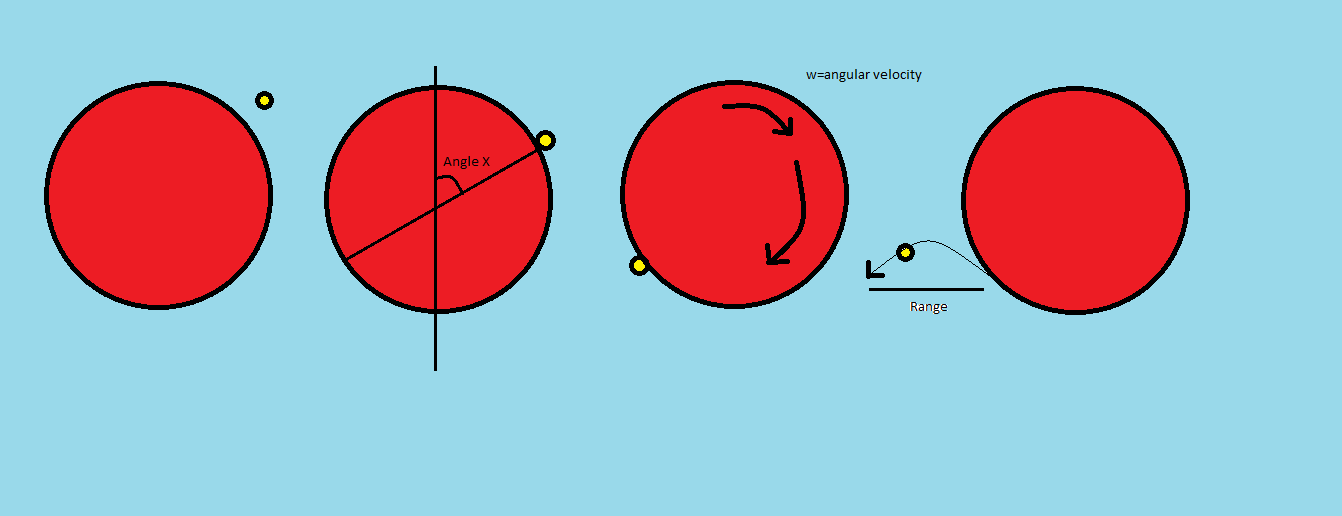# A giant disc and tiny ballsSuppose a very massive disc is there, which was initially at Rest with its centre pivoted in to a vertical wall, And i drop balls upon it from rest/negligible initial velocity the balls stick to the disc at angle 'x' with the vertical, the disc rotates along with them and the balls leave the disc tangentially flying off at the point diametrically opposite And several several such balls are dropped in similar fashion continuously without breaks, "All collisions are inelastic", and "balls are very light compared to disc" . Then it turns out that the disc after some time reaches a saturation angular velocity, that is the process becomes smooth and angular acceleration of disc ceases,

Then find the value of 'sin(x)' (the angle) for which the balls leaving tangentially will reach maximum range on ground when the disc has attained the constant angular velocity

Refer to figure for getting a clearer picture, only one ball has been shown for clarity

NOTE

1) The problem is simpler than it looks

2) Calculus not required, but if you do it using calculus, do share your method

3) Carefully observe and ask yourself, why does the disc rotate, who provides the energy, and where does the system ever lose energy to attain saturation, and if so what should be the criteria satisfied for reaching that state

×